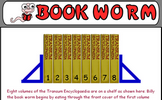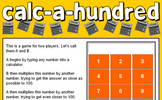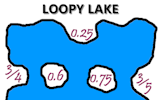# Decimals

### Term 2 starting in week 1 :: Estimated time: 2 weeks

• Decimals up to 2 decimal places (review)
• Understand thousandths (review)
• Three decimal places
• Multiply by 10, IOO and 1,000
• Divide by 10, 100 and 1,000
• Multiply decimals by integers
• Divide decimals by integers
• Division to solve problems
• Decimals as fractions
• Fractions to decimals (1)
• Fractions to decimals (2)

This page should remember your ticks from one visit to the next for a period of time. It does this by using Local Storage so the information is saved only on the computer you are working on right now.

## Lesson Starters

Here are some suggestions for whole-class, projectable resources which can be used at the beginnings of each lesson in this block.

### 1st Lesson#### Divided Age

How old is a person if when her age is divided by certain numbers, the calculator display ending are as shown.

### 2nd Lesson#### Book Worm

Work out the distance the book worm needs to eat through to get to the back cover of the last book.

### 3rd Lesson#### Calc-A-Hundred

A game for two players requiring a calculator and thinking skills.

### 4th Lesson#### Loopy Lake

Match the fractions to their decimal equivalents and join them with lines that do not cross.

### 5th Lesson#### Noodlewhack

Divide one whole number by another on your calculator. If the answer has one digit repeated but no other digits you have a Noodlewhack.

### 6th Lesson#### Refreshing Revision

It is called Refreshing Revision because every time you refresh the page you get different revision questions.

Some of the Starters above are to reinforce concepts learnt, others are to introduce new ideas while others are on unrelated topics designed for retrieval practice or and opportunity to develop problem-solving skills.

White Rose ResourcesEnd of block assessments provide a quick progress check at the end of each block of learning to make sure students have understood the content covered. This Scheme of Learning was produced by White Rose Maths and is used here with permission granted on 30th June 2021.For All: